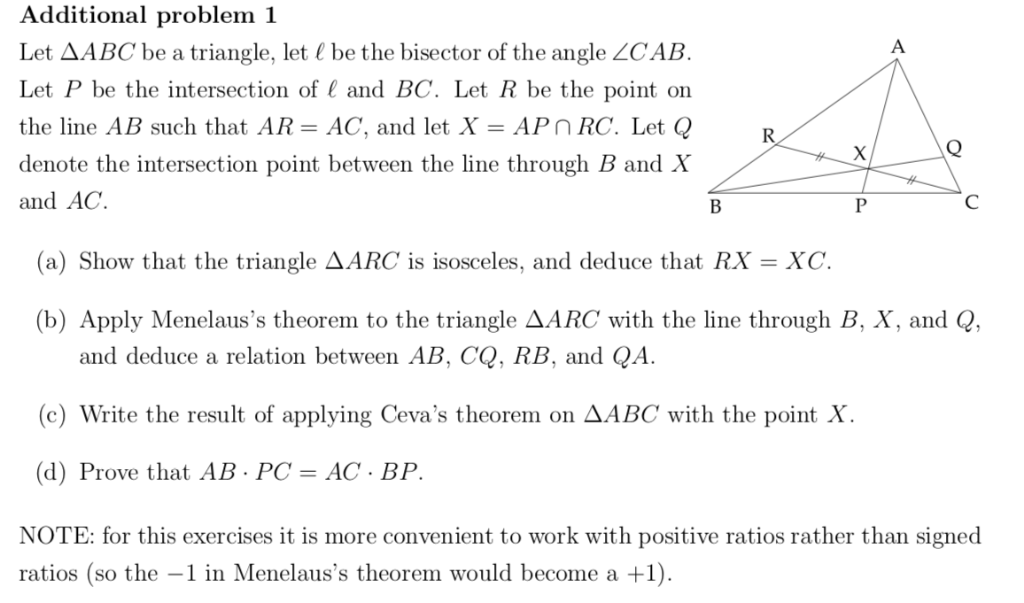# Additional problem 1 Let AABC be a triangle, let be the bisector of the angle ZCAB Let P be the intersection of and BC. Let R be the point on the line AB such that AR-AC, and let X-APnRC. Let Q denot... related homework questions

• #### Additional problem 1 Let AABC be a triangle, let be the bisector of the angle ZCAB Let P be the intersection of and BC. Let R be the point on the line AB such that AR-AC, and let X-APnRC. Let Q denot...Additional problem 1 Let AABC be a triangle, let be the bisector of the angle ZCAB Let P be the intersection of and BC. Let R be the point on the line AB such that AR-AC, and let X-APnRC. Let Q denote the intersection point between the line through B and X and AC. (a) Show that the triangle AARC...

• #### It’s review question, I need this as soon as possible. Thank you 3) For thè diferential equation: (a) The point zo =-1 is an ordinary point. Compute the recursion formula for the coefficients of...It’s review question, I need this as soon as possible. Thank you 3) For thè diferential equation: (a) The point zo =-1 is an ordinary point. Compute the recursion formula for the coefficients of the power series solution centered at zo- -1 and use it to compute the first three nonzero terms of the power series when -1)-s and v(-1)-0....

• #### Dont copié formé thé book oh ya dont copié formé thé book cause you Oiil inde up being triste soi remembré not toi copié frome thé book oh ya

Dont copié formé thé book oh ya dont copié formé thé book cause you Oiil inde up being triste soi remembré not toi copié frome thé book oh ya!translation in english please!

• #### A triangle has corners at points A, B, and C. Side AB has a length of #38 #. The distance between the intersection of point A's angle bisector with side BC and point B is #8 #. If side AC has a length of #46 #, what is the length of side BC?

Free Homework App